# # 索引失效有哪些？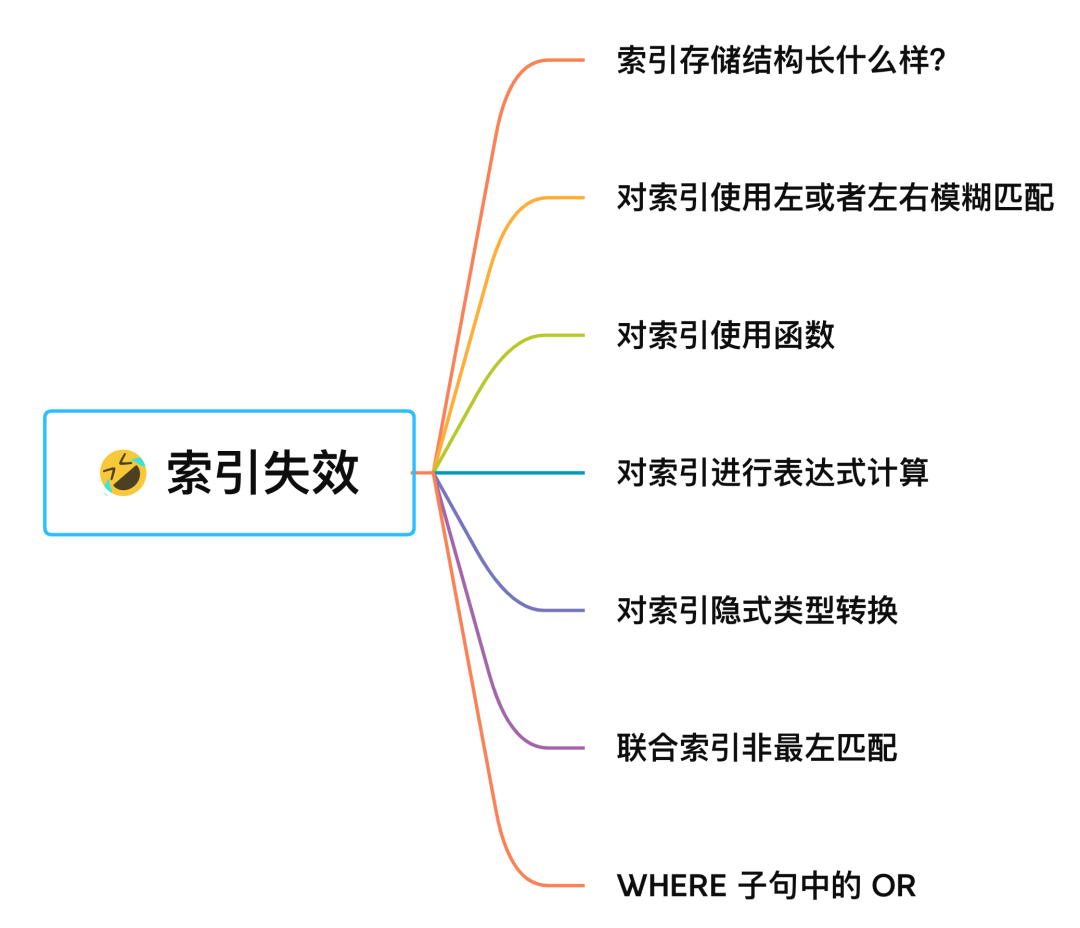## # 索引存储结构长什么样？

MySQL 默认的存储引擎是 InnoDB，它采用 B+Tree 作为索引的数据结构，至于为什么选择 B+ 树作为索引的数据结构 ，详细的分析可以看我这篇文章：为什么 MySQL 喜欢 B+ 树？ (opens new window)

MySQL 的 MyISAM 存储引擎支持多种索引数据结构，比如 B+ 树索引、R 树索引、Full-Text 索引。MyISAM 存储引擎在创建表时，创建的主键索引默认使用的是 B+ 树索引。

• InnoDB 存储引擎：B+ 树索引的叶子节点保存数据本身；
• MyISAM 存储引擎：B+ 树索引的叶子节点保存数据的物理地址；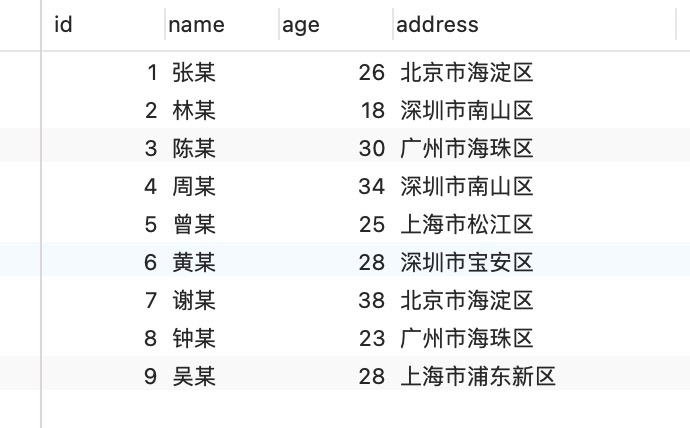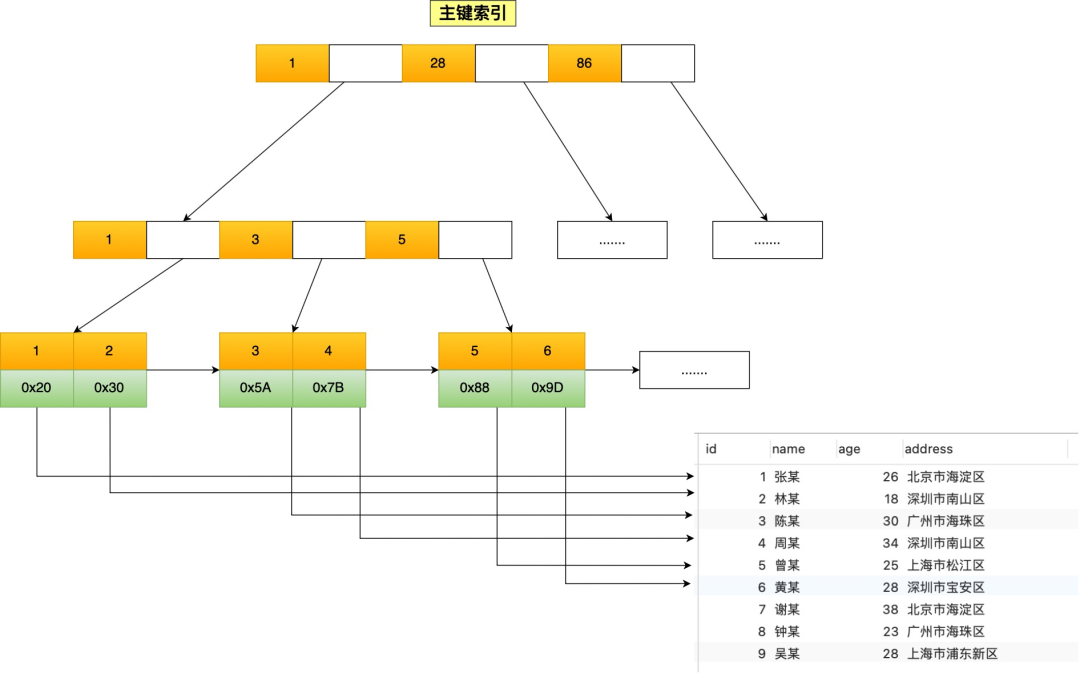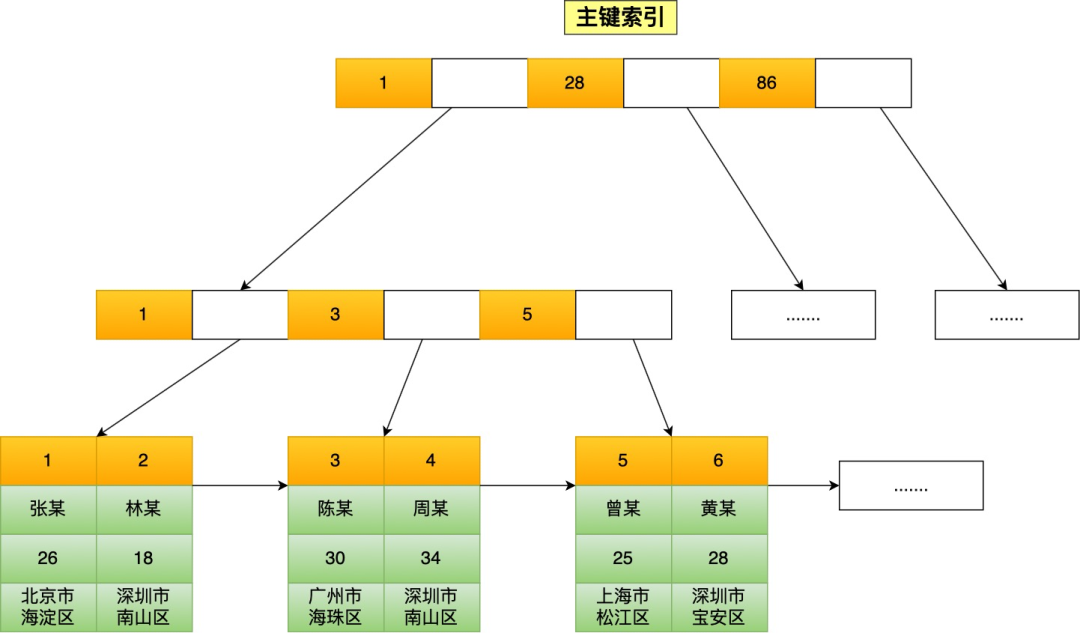InnoDB 存储引擎根据索引类型不同，分为聚簇索引（上图就是聚簇索引）和二级索引。它们区别在于，聚簇索引的叶子节点存放的是实际数据，所有完整的用户数据都存放在聚簇索引的叶子节点，而二级索引的叶子节点存放的是主键值，而不是实际数据。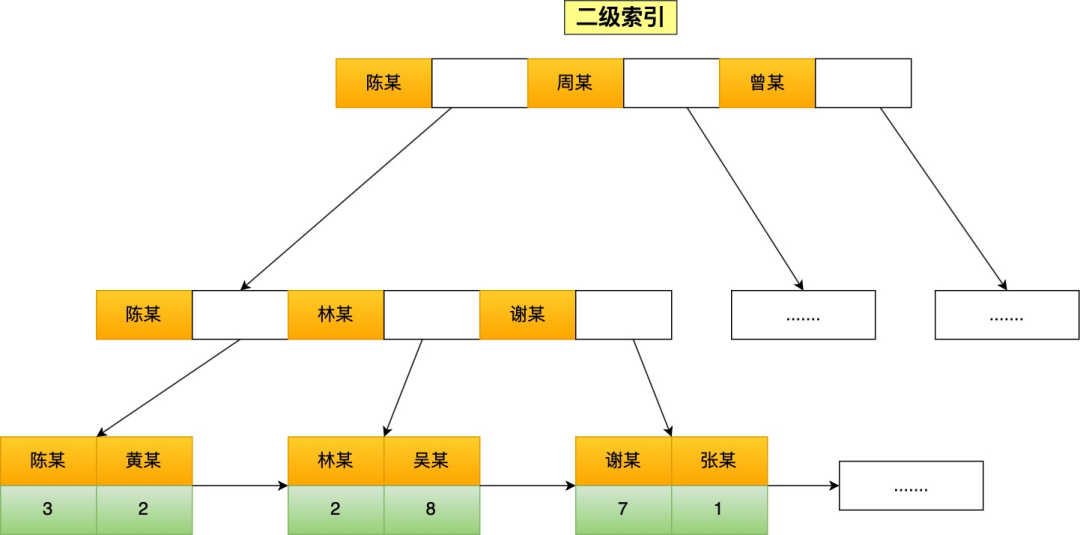``````// id 字段为主键索引
select * from t_user where id=1;
``````

• 先在「二级索引」的 B+ 树找到对应的叶子节点，获取主键值；
• 然后用上一步获取的主键值，在「聚簇索引」中的 B+ 树检索到对应的叶子节点，然后获取要查询的数据。

``````// name 字段为二级索引
select * from t_user where name="林某";
``````

``````// name 字段为二级索引
select id from t_user where name="林某";
``````

## # 对索引使用左或者左右模糊匹配

``````// name 字段为二级索引
select * from t_user where name like '%林';
``````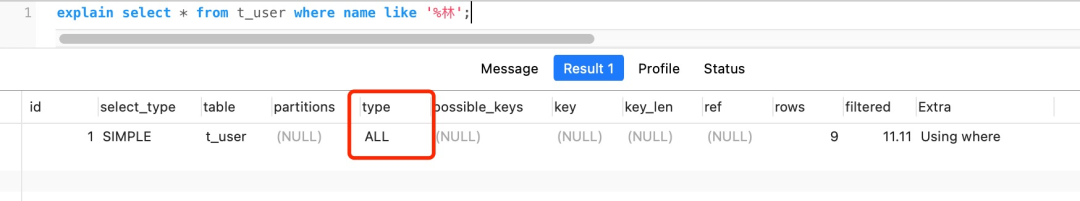``````// name 字段为二级索引
select * from t_user where name like '林%';
``````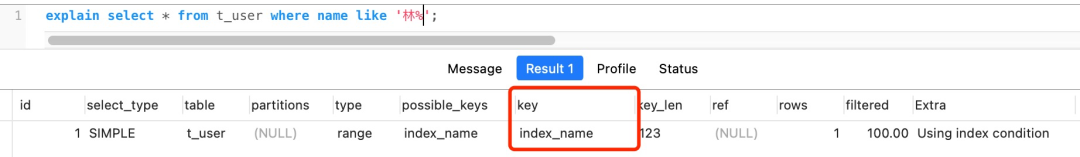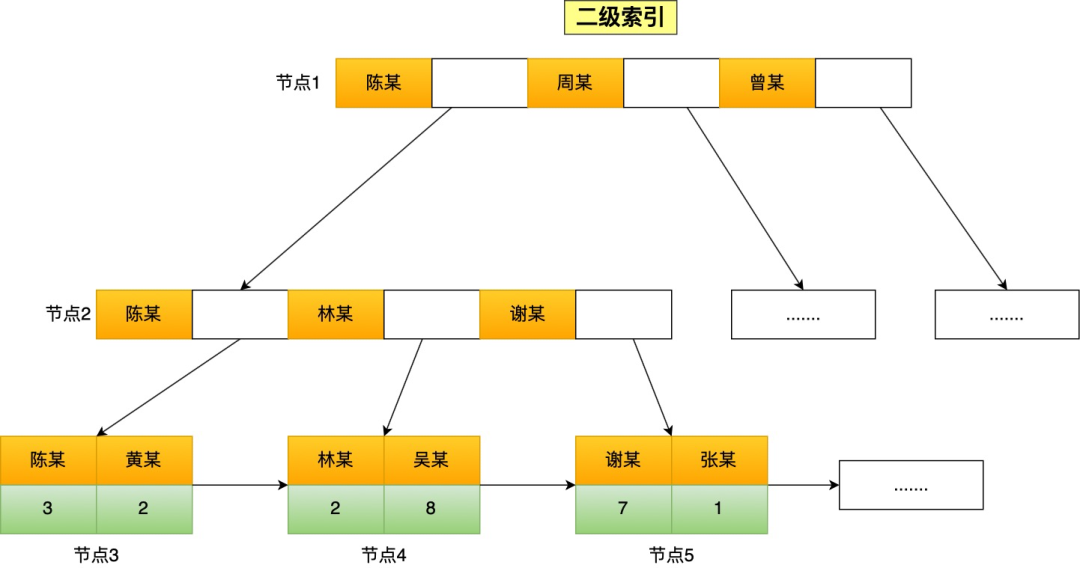• 首节点查询比较：林这个字的拼音大小比首节点的第一个索引值中的陈字大，但是比首节点的第二个索引值中的周字小，所以选择去节点2继续查询；
• 节点 2 查询比较：节点2的第一个索引值中的陈字的拼音大小比林字小，所以继续看下一个索引值，发现节点2有与林字前缀匹配的索引值，于是就往叶子节点查询，即叶子节点4；
• 节点 4 查询比较：节点4的第一个索引值的前缀符合林字，于是就读取该行数据，接着继续往右匹配，直到匹配不到前缀为林的索引值。

## # 对索引使用函数

``````// name 为二级索引
select * from t_user where length(name)=6;
``````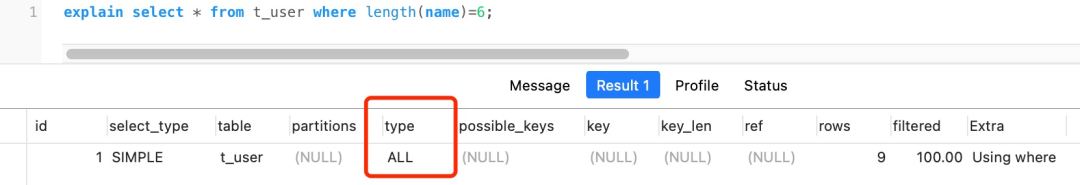``````alter table t_user add key idx_name_length ((length(name)));
``````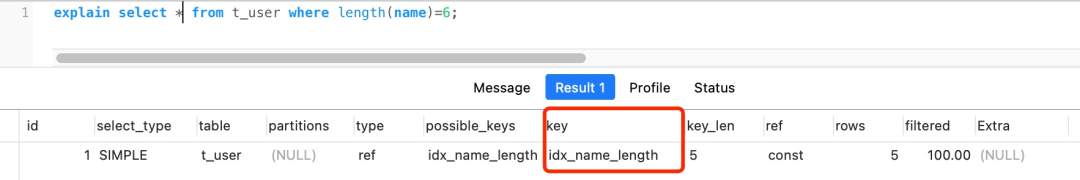## # 对索引进行表达式计算

``````explain select * from t_user where id + 1 = 10;
``````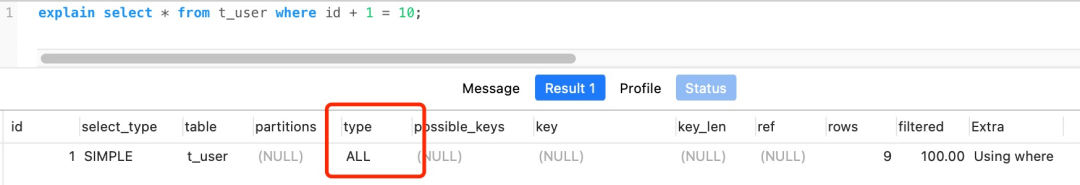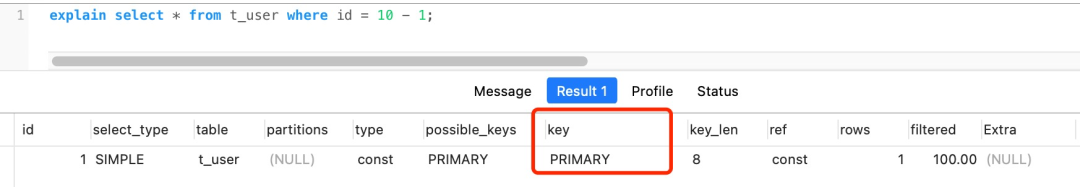## # 对索引隐式类型转换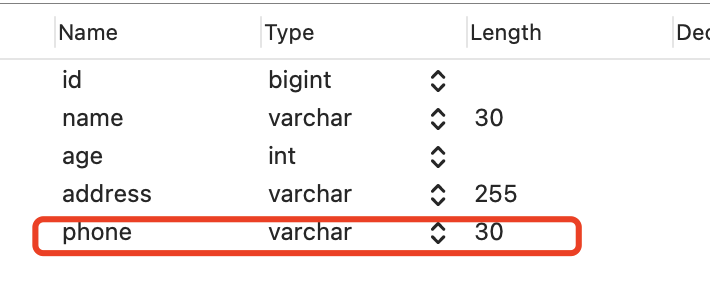``````select * from t_user where phone = 1300000001;
``````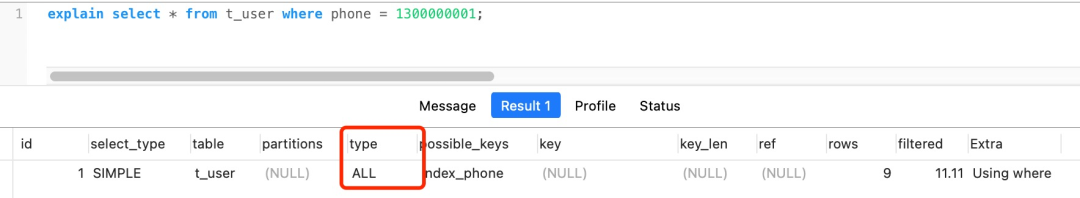`````` explain select * from t_user where id = '1';
``````• 如果规则是 MySQL 会将自动「字符串」转换成「数字」，就相当于 select 10 > 9，这个就是数字比较，所以结果应该是 1；
• 如果规则是 MySQL 会将自动「数字」转换成「字符串」，就相当于 select "10" > "9"，这个是字符串比较，字符串比较大小是逐位从高位到低位逐个比较（按ascii码） ，那么"10"字符串相当于 “1”和“0”字符的组合，所以先是拿 “1” 字符和 “9” 字符比较，因为 “1” 字符比 “9” 字符小，所以结果应该是 0。``````//例子一的查询语句
select * from t_user where phone = 1300000001;
``````

``````select * from t_user where CAST(phone AS signed int) = 1300000001;
``````

``````//例子二的查询语句
select * from t_user where id = "1";
``````

``````select * from t_user where id = CAST("1" AS signed int);
``````

## # 联合索引非最左匹配

• where a=1；
• where a=1 and b=2 and c=3；
• where a=1 and b=2；

• where b=2；
• where c=3；
• where b=2 and c=3；

MySQL 5.5 的话，前面 a 会走索引，在联合索引找到主键值后，开始回表，到主键索引读取数据行，Server 层从存储引擎层获取到数据行后，然后在 Server 层再比对 c 字段的值。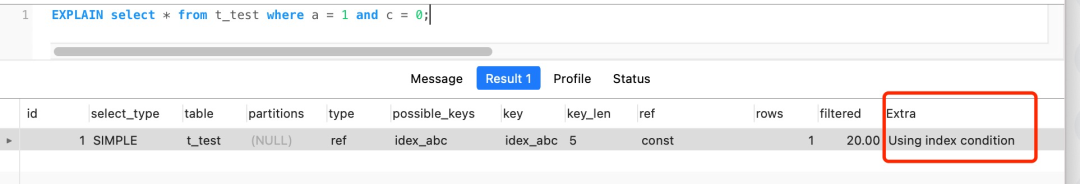## # WHERE 子句中的 OR

``````select * from t_user where id = 1 or age = 18;
``````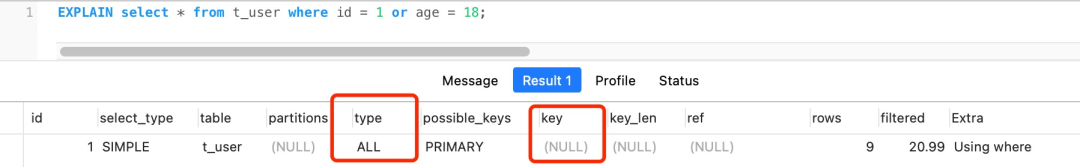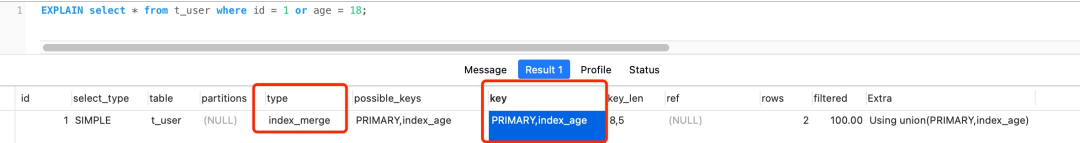## # 总结

• 当我们使用左或者左右模糊匹配的时候，也就是 `like %xx` 或者 `like %xx%`这两种方式都会造成索引失效；
• 当我们在查询条件中对索引列使用函数，就会导致索引失效。
• 当我们在查询条件中对索引列进行表达式计算，也是无法走索引的。
• MySQL 在遇到字符串和数字比较的时候，会自动把字符串转为数字，然后再进行比较。如果字符串是索引列，而条件语句中的输入参数是数字的话，那么索引列会发生隐式类型转换，由于隐式类型转换是通过 CAST 函数实现的，等同于对索引列使用了函数，所以就会导致索引失效。
• 联合索引要能正确使用需要遵循最左匹配原则，也就是按照最左优先的方式进行索引的匹配，否则就会导致索引失效。
• 在 WHERE 子句中，如果在 OR 前的条件列是索引列，而在 OR 后的条件列不是索引列，那么索引会失效。